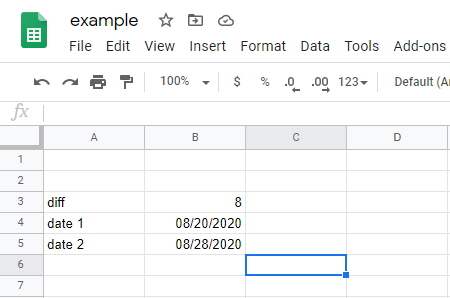Home
IT Knowledge
Inspiration
Languages
EN

# Google excel - calculate difference in days between two dates

12 points
Created by: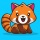Bartek
2110

In this short article, I would like to share how to count days between two dates in google sheets.

Let's say we have 2 dates:

• 08/20/2020
• 08/28/2020

## Example 1:

The difference is quite small, only 8 days.

We can use below formula to calculate difference in days between two dates:

``=DATEDIF("08/20/2020", "08/28/2020", "d")``

We can take a look at below screenshot, to see how it will look like in practice: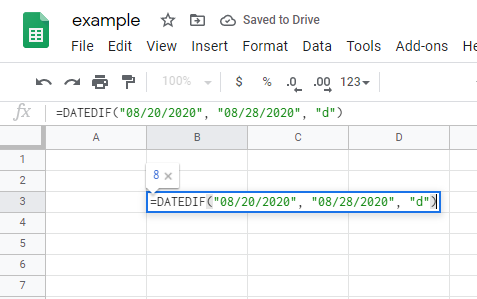As the result we will get: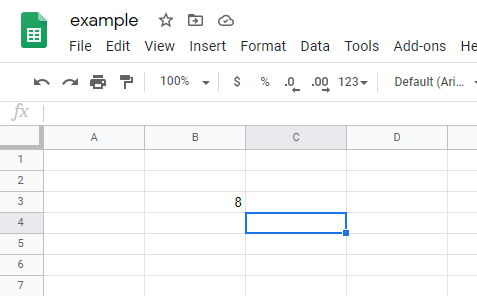## Exampl 2:

This is similar to example 1, but we can also use fields in online excel like this:

``=DATEDIF(B4, B5, "d")``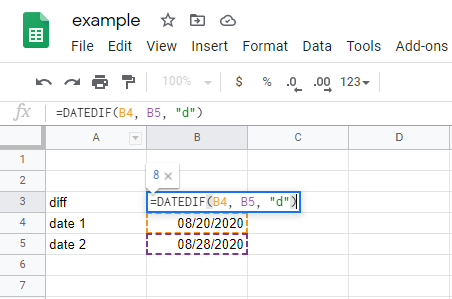As the result we will get: